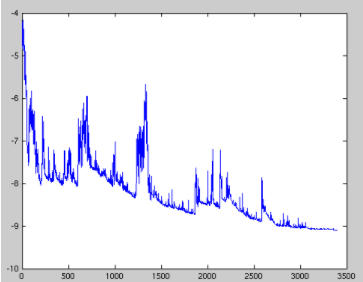# 深度学习-常见优化器算法

## Batch Gradient Descent(BGD)

BGD 采用整个训练集的数据来计算 cost function 对参数的梯度：

$θ = θ - \eta \cdot \bigtriangledown_{θ}J(θ)$
for i in range(nb_epochs):
params = params - learning_rate * params_grad


Batch gradient descent 对于凸函数可以收敛到全局极小值，对于非凸函数可以收敛到局部极小值。

## Stochastic Gradient Descent(SGD)

$θ = θ - \eta \cdot \bigtriangledown_{θ}J(θ;x^{(i)},y^{(i)})$
for i in range(nb_epochs):
np.random.shuffle(data)
for example in data:
params = params - learning_rate * params_gradSGD对所有参数更新时应用同样的 learning rate，如果我们的数据是稀疏的，我们更希望对出现频率低的特征进行大一点的更新。LR会随着更新的次数逐渐变小。

## Mini-Batch Gradient Descent(MBGD)

MBGD 每一次利用一小批样本，即 n 个样本进行计算，这样它可以降低参数更新时的方差，收敛更稳定，另一方面可以充分地利用深度学习库中高度优化的矩阵操作来进行更有效的梯度计算。

$θ = θ - \eta \cdot \bigtriangledown_{θ}J(θ;x^{(i:i+n)},y^{(i:i+n)})$

for i in range(nb_epochs):
np.random.shuffle(data)
for batch in get_batches(data, batch_size=50):
params = params - learning_rate * params_grad


MBGD不能保证很好的收敛性，learning rate 如果选择的太小，收敛速度会很慢，如果太大，loss function 就会在极小值处不停地震荡甚至偏离。（有一种措施是先设定大一点的学习率，当两次迭代之间的变化低于某个阈值后，就减小 learning rate，不过这个阈值的设定需要提前写好，这样的话就不能够适应数据集的特点。）对于非凸函数，还要避免陷于局部极小值处，或者鞍点处，因为鞍点周围的error是一样的，所有维度的梯度都接近于0，SGD 很容易被困在这里。（会在鞍点或者局部最小点震荡跳动，因为在此点处，如果是训练集全集带入即BGD，则优化会停止不动，如果是mini-batch或者SGD，每次找到的梯度都是不同的，就会发生震荡，来回跳动。）